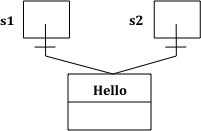4.4. String Equality¶

When the operator `==` is used with object variables it returns true when the two variables refer to the same object. With strings this happens when one string variable is set to another or when strings are set to the same string literal.

If you run the following, what will be printed?

It will print `Bye` since s3 has been assigned to a copy of the value in s2 which is an object reference to the String object that has the characters “Bye” in it. In addition, `s2 == s3` will be true since the two variables refer to the same object. Also, `s2.equals(s3)` will also be true, again since the two variables refer to the same object, of course the characters will be the same.Figure 1: Several String variables with references to objects of the String class.

Note

Use `equals` to test if two strings have the same characters in the same order. Only use `==` to test if two strings refer to the same object. Most of the time you will want to use `equals` and not `==` with strings.

4.4.1. Using the New Keyword¶

If you use the `new` keyword to create a string it will create a new string object. So, even if we create two string objects with the same characters using the new operator they will not refer to the same object. What will the following print?

Since we used the `new` keyword two different String objects will be created that each have the characters `Hello` in them. So `s1 == s2` will be false since they don’t refer to the same object, but `s1.equals(s2)` is true since the two different object contain the same characters in the same order.Figure 2: Two string variables and two string objects that contain the same characters in the same order.

4.4.2. Using String Literals¶

What do you think the following code will print? Run it to check.

Since we used string literals this time rather than the `new` keyword, the Java run-time will check if that string literal already exists as an object in memory, and if so reuse it. So `s1` and `s2` will refer to the same string object. That means that both `==` and `equals` will be true.Figure 3: Two string variables that refer to the same string literal.

4-3-1: Which of the following is true after the code executes?

```String s1 = new String("hi");
String s2 = "bye";
String s3 = "hi";
s2 = s1;
```
• s1 == s2 && s1 == s3
• Do s1 and s3 refer to the same object?
• s1 == s2 && s1.equals(s3)
• Yes s2 was set to refer to the same object as s1 and s1 and s3 have the same characters.
• s1 != s2 && s1.equals(s3)
• Did you miss that s2 was set to refer to the same object as s1?

4-3-2: Which of the following is true after the code executes?

```String s1 = "hi";
String s2 = "bye";
String s3 = "hi";
```
• s1 == s2 && s1 == s3
• Do s1 and s2 refer to the same object?
• s2.equals(s3) && s1.equals(s3)
• Does s2 have the same characters as s1 or s3?
• s1 != s2 && s1 == s3
• Because you used the same string literal s1 and s3 will refer to the same object. Since s1 and s2 refer to different string literals they do not refer to the same object.

4-3-3: Which of the following is true after the code executes?

```String s1 = "hi";
String s2 = "bye";
String s3 = new String("hi");
```
• s1 == s3 && s1.equals(s3)
• Since s3 uses the new operator it will not refer to the same object as s1.
• s2.equals(s3) && s1.equals(s3)
• Do s2 and s3 have the same characters in the same order?
• !(s1 == s2) && !(s1 == s3)
• All of the variables refer to different objects. But, s1.equals(s3) would be true since they have the same characters in the same order.
Next Section - 4.5. String Operators - Concatenation